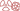THE PHENOMENON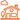IN DAILY LIFE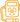QUESTION OF DOSES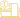AT THE DOCTOR’S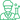At the laboratory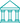At the museum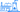NUCLEAR ENERGY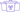The aim of this web site is to explain radioactivity and its applications to the general public.

# Beta spectrum

## Beta electrons do not have a unique characteristic energy

Three particles, the recoil nucleus, the beta electron and its antineutrino ,share the energy released in a beta decay. The nucleus, which is extremely heavy compared to the two other two, takes a negligible part of the available energy, which is shared in practice between the electron and the antineutrino. In average, the electron carries a little less than 50% of this energy, and the antineurino a little more than 50 %.

In the (rare) case of a beta-plus decay, it is the positron which plays the part of the electron, the neutrino that of the antineutrino, but the scenario and the sharing are the same.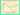Beta spectrum : a sharing of energy
In a beta decay like that of a bismuth-210 nucleus, the decay energy is shared between the nucleus, an electron and an antineutrino. The nucleus, much more massive than the two others (its mass is 320 000 times the mass of the electron) takes away a negligible amount of kinetic energy. The energy is therefore shared between the electron and the antineutrino. The antineutrino escaping detection, one observe only the beta electron with a variable energy. The figure display the characteristic energy repartition of beta electrons – the beta spectrum – of the bismuth-decay

The distribution of the beta electron energy – called beta spectrum – is characteristic. As the kinetic energy of the emitting nucleus in negligible, it is the electron and the antineutrino that share the decay energy in variable proportions. The electron energy is maximal when it carries all the decay energy. It becomes null, when it is the antineutrino.

The shape of the beta spectrum is asymetrical with a predominance of low energy electrons. The antineutrino whose mass is quasi-null travels at the speed of light and carries more kinetic energy than the electron which is heavy in comparison, despite its extreme lightness. Accordingly few beta electrons approach the maximum energy allowed, while the majority of them have relatively low energies.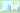Examples of average beta energies
Since the energy of beta electrons emitted by a radionucleide is not unique, one compares generally radionucleides by their average beta energies. These energies vary considerably : for instance, the average energy of beta emitted by tritium is a hundred times lower than that of the phosphorus-32 beta. The average energies are below 1 MeV, much lower than those of alpha particles (usually above 4 MeV).

The prevalence of low energy beta electrons is beneficial for radiological protection, because low energy electrons are easier to stop. In radiological protection, one is more interested in the average energy of electrons than by their maximum energy. This average energy varies within wide proportions, ranging from 5.69 keV in the case of tritium to 695 keV in the case of phosphorus-32 a powerful beta emitter.

Compared to alpha particles, the energies of beta electrons are much lower, below 1 MeV  in most of the cases while the energies of alpha particles are always above 4000 keV (4 MeV). The lifetimes (half-lives) are much shorter, with the exception of potassium-40.

A beta decay often comes with the emission of a gamma-ray due to the desexcitation of the nucleus. This emission decreases the energy to be shared between the electron and the antineutrino. For instance, the available energy in the caesium-137 beta decay is 1176 keV, but in 95 % of the cases it is accompanied by the emission of a characteristic 662 kev gamma in which case the available energy is only 514 keV. The observed spectrum beta becomes the sum, in the proportions of 5% and 95%, of the two spectra corresponding to the modes without or with gamma.

In addition, it may happen – this is the phenomenon of internal conversion- that gamma transmit their energy to an electron of the atom. These electrons are not strictly speaking beta electrons and have unique characteristic energies.

NEXT : The electron-neutrino
NEXT : The positronTHE PHENOMENONIN DAILY LIFEQUESTION OF DOSESAT THE DOCTOR’SAt the laboratoryAt the museumNUCLEAR ENERGY